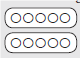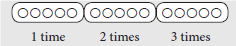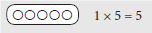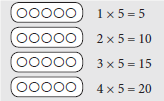# Lesson Notes By Weeks and Term - Primary 2

Multiplication (5 times table)

TERM: 2nd Term

WEEK: 12

CLASS: Primary 2

AGE: 7 years

DURATION: 5 periods of 40 minutes each

DATE:

SUBJECT: Mathematics

TOPIC: Multiplication (5 times table)

SPECIFIC OBJECTIVES: At the end of the lesson, the pupils should be able to

1. Solve multiplication number sentences.
2. Learn the 5 times table – building it up.
3. Consolidate knowledge of the 5 times table.
4. Write multiplication number sentences.

INSTRUCTIONAL TECHNIQUES: Explanation, question and answer, demonstration, practical

INSTRUCTIONAL MATERIALS: Bottle tops, Multiplication cards(5x)

PERIOD 1: Assessment

PRESENTATION

TEACHER’S ACTIVITY

PUPIL’S ACTIVITY

STEP 1

ORAL

ASSESSMENTS

The teacher asks questions on mental maths treated so far

Pupils respond and participate

STEP 2

DISCUSSION

The teacher discusses all the methods used by some learners in the oral assessments(some of the questions are solved on the board by the learners) and addresses any misconceptions that may have risen

Pupils pay attention and participate

STEP 3

WRITTEN ASSESSMENTS

1. Complete the table

 Groups Multiplication Repeated addition 1. 4 groups of 5 4 x 5 = 20 2. ___ groups of ___ 9 x 2 = 3. 7 groups of 3 7 x 3 = 4. ___ groups of __ 5 x 4 = 5. 10 groups of 3 10 x 3 =

2 Draw a diagram to calculate the answer. 7 × 4 = ______

Pupils attempt their class work

STEP 4

SUMMARY

The teacher marks the written assessments, corrects were necessary and commends the pupils

PERIOD 2: Multiplication number sentences

 PRESENTATION TEACHER’S ACTIVITY PUPIL’S ACTIVITY STEP 1MENTAL MATHS The teacher begins the lesson with some mental calculationsCalclate1 2 + 2 + 2 =  2 5 + 5 = 3 4 + 4 + 4+ 4+ 4 =  4 3 + 3 + 3 =5 2 + 2 + 2 + 2+ 2 + 2 =  6 3 + 3 + 3 + 3 + 3 + 3 =7 5 + 5 + 5 + 5 =8 2 + 2 + 2 + 2 + 2 + 2 + 2 =9 4 + 4 + 4 + 4 + 4 + 4 + 4 =10 5 + 5 + 5 + 5 + 5 + 5 + 5 = Pupils respond and participate STEP 2CONCEPTDEVELOPMENT The teacher• Writes the following word problem on the board.Tacha has 5 apples.Janice has 2 times as many apples as Tacha.How many apples does Jabu have?• Reads the problem.• Asks: What is the story about? (apples)• Asks: What numbers do you see in the story? (5 and 2)• Underlines these numbers.• Asks: What is the question? (How many apples does Janice have?)• Underlines the question with a wavy line.• Lets the learners read the problem until they read it fluently.• Draws the diagram as follows.• Explains: When you say 2 times as many, it means if Tacha has 1 group of 5 apples then Janice has 2 groups of 5 apples.• Asks: How do we write the number sentence for Janice’s apples? (2 × 5 = 10, 10 apples.)Why? (Because Janice has 2 groups of 5 apples.)• Lets the learners draw the diagram and write the number sentence with its answer in their classwork books.• Lets a learner write his/her multiplication number sentence on the board for correction. CLASS ACTIVITYThe teacher• Writes the following word problem on the board. I have a 4 m piece of string.How long would the string be if it was 3 times longer? • Reads the problem.• Asks: What is the story about? (String)• Asks: What numbers do you see in the story? (4 and 3)• Underlines these numbers.• Asks: What is the question? (How long would the string be if it was 3 times longer?)• Underlines the question with a wavy line.• Lets the learners read the problem until they read it fluently.• Draws the diagram shown below on the board.• Asks: How do we write a number sentence for this story? (3 × 4 = __) Why? (Because there are 3 groups of 4 circles).• Learners solve the problem. Let the learners write the number sentence with the answer in their classwork books. (3 × 4 = 12, 12m)• Lets a learner write his/her multiplication number sentence on the board for correction.If some learners still write the number sentence as repeated addition, let them write the multiplication number sentence under the repeated addition. Gradually they need to shift from repeated addition to multiplication. Pupils pay attention and participate STEP 3CLASS-WORK Draw a diagram to calculate the answer.1 What is 4 times 5? _____2 What is 3 times 4? _____3 What is 6 times 3? _____4 What is 8 times 2? _____5 What is 7 times 5? _____6 What is 9 times 4? _____ Pupils attempt their class work STEP 4HOME-WORK Draw a diagram to calculate the answer.1 What is 5 times 3? ______2 What is 7 times 2? ______ The pupils writes it in their homework book STEP 5SUMMARY The teacher summarizes by reminding the pupils that in this lesson we have learnt to multiply using number sentences. She marks their class works, makes corrections where necessary and commends them positively

PERIOD 3: 5 times table

PRESENTATION

TEACHER’S ACTIVITY

PUPIL’S ACTIVITY

STEP 1

MENTAL MATHS

The teacher begins the lesson with some mental calculations

Count forwards and backwards in 5s up to 50.

Pupils respond and participate

STEP 2

CONCEPT

DEVELOPMENT

The teacher

• Writes the word problem on the board.

There are 5 balls in a bag.

How many balls are there in 1 bag?

• Draws the diagram below on the board while learners place 5 bottle tops horizontally across their desks.Asks: How can we write the multiplication sentence for this drawing? (1 × 5 = 5)

Why? (Because there is 1 group of 5 bottle tops.)

How many balls are there? (5 balls)

Lets the learners draw 5 circles in a horizontal line and write the multiplication number sentence 1 × 5 = 5 next to the drawing in their classwork books.

Says: This is 1 times 5, writing 1 × 5 = 5 next to the drawing on the board.

Continues this activity with 2 bags, 3 bags, and 4 bags.

Draws the diagrams underneath each other and write the number sentence next to the drawing each time.Lets the learners read the number sentence every time and draw the same diagram and writes the multiplication number sentence in their classwork books.

CLASS ACTIVITY

The teacher

• Asks some learners to come to the board to complete the 5 times table up to 10 times 5, following the diagram in Activity 1.

5 × 5 = 25, 6 × 5 = 30 until you get to 10 × 5 = 50.

• Once all the number sentences are written on the board, asks: Do you notice anything about the answers to these number sentences? (They end in 0 or 5, the answers increase by 5 each time).

• Says: We have found a pattern in the five times table.

• Lets the learners continue to complete the 5 times table by drawing in their classwork books.

Pupils pay attention and participate

STEP 3

CLASS-WORK

NOTE: Learners may use bottle tops to help them do this activity.

The Grade 2 class is going to the zoo. Each car can take 5 children. How many children can

go to the zoo each time?

Complete the table.

 Cars Number sentence Answer 1 1 car 1 x 5 = 5 5 children 2 2 cars 3 3 cars 4 4 cars 5 5 cars 6 6 cars 7 7 cars 8 8 cars 9 9 cars

Pupils attempt their class work

STEP 4

HOME-WORK

Complete the number sequences counting in 5s:

1 0, 5, 10, ____, 20, ____, ____, 35, 40, ____, 50.

2 50, 45, ____, 35, 30,____, 20, 15, ____, 5, 0.

The pupils writes it in their homework book

STEP 5

SUMMARY

The teacher summarizes by reminding the pupils that in today’s lesson we have learnt the 5 times table.

PERIOD 4: 5 times table

PRESENTATION

TEACHER’S ACTIVITY

PUPIL’S ACTIVITY

STEP 1

MENTAL MATHS

The teacher begins the lesson with some mental calculations

What is the multiplication number sentence?

 Repeated addition Multiplication 1 5 + 5 2 5 + 5 + 5 + 5 + 5 + 5 3 5 + 5 + 5 + 5 + 5 + 5 + 5 + 5 4 5 + 5 + 5 + 5 5 5 + 5 + 5 + 5 + 5 6 5 + 5 + 5 + 5 + 5 + 5 + 5 7 5 + 5 + 5 8 5 + 5 + 5 + 5 + 5 + 5 + 5 + 5 + 5 + 5 9 5 + 5 + 5 + 5 + 5 + 5 + 5 + 5 + 5

Pupils respond and participate

STEP 2

CONCEPT

DEVELOPMENT

The teacher

Gives each pair of learners a set of multiplication cards (5 times table).

The learners should cut up the cards. They should write the answers on the back of each card, to prepare them for use in this and other lessons. Make sure that they write the correct answers on the backs of the cards.

The learners should put their cards in order from 1 × 5 = 5 to 10 × 5 = 50 on their desks.

Learners turn their cards over to show the answer side up.

The teacher says a multiplication problem from the 5 times table.

Learners grab the card with the correct answer.

CLASS ACTIVITY

The teacher

• Using the 5 times table cards play the following games.

• Explains one game at a time.

When the fast learners become tired of the first game, let them move on to the next game.

You should walk around the class and make sure all of the learners a playing a game and having fun learning the 5 times table.

• Helps learners who have not understood the rules of the games if necessary.

Game 1

1 One learner picks up one card at a time and reads it out loud.

2 The other learner gives the answer.

3 Learners take turns to ask and answer questions, checking the answers at the back of the card each time.

Game 2

1 Both learners draw a card from the pile.

2 They say the answer to each other.

3 The learner who gets the bigger answer is the winner.

4 When you have completed the cards, you can play the game again but this

time the learner who gets the smaller answer is the winner.

Game 3

1 Lay all the cards out with the answers facing up.

2 Pick a card and say the multiplication number sentence for which the

number you chose is the answer.

3 Take turns.

Pupils pay attention and participate

STEP 3

CLASS-WORK

Complete the table. Write repeated addition as multiplication.

 Multiplication Repeated addition Answer 1 6 x 5 = 2 3 x 5 = 3 5 + 5 4 7 x 5 =

Pupils attempt their class work

STEP 4

HOME-WORK

Complete the table. Write repeated addition as multiplication.

 Multiplication Repeated addition Answer 1 2 x 5 = 2 5 x 5 = 3 5 + 5 + 5 + 5 4 8 x 5 =

The pupils writes it in their homework book

STEP 5

SUMMARY

The teacher summarizes by reminding the pupils that in today’s lesson we have learnt the five times table.

She marks their class works, makes corrections where necessary and commends them positively

PERIOD 5: Weekly Test/consolidations

TEACHER’S ACTIVITY: The teacher revises all the concepts treated from period 1-4 and gives the pupils follow through exercises, quiz and tests. She marks the exercises, makes corrections and commends the pupils positively.

PUPIL’S ACTIVITY: The pupils work on the worksheets and exercises give by the teacher individually

CONSOLIDATION

1 Complete the table. Write repeated addition as multiplication

 Multiplication Repeated addition Answer 1. 2 x 5 = 5 + 5 = 10 2.  3 x 5 3. 4 x 5 4. 5 x 5 5. 6 x 5 6. 7 x 5 7. 8 x 5 8. 9 x 5 9. 10 x 5

2 Play the 5 times table card game. Your teacher will explain the rules.